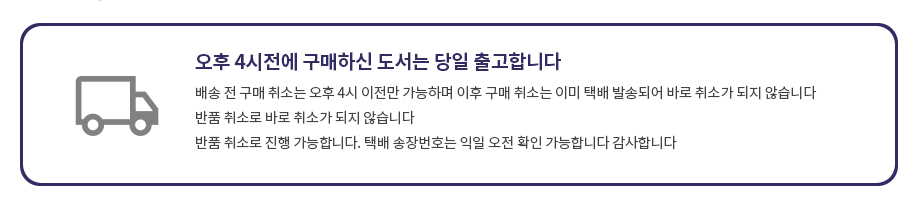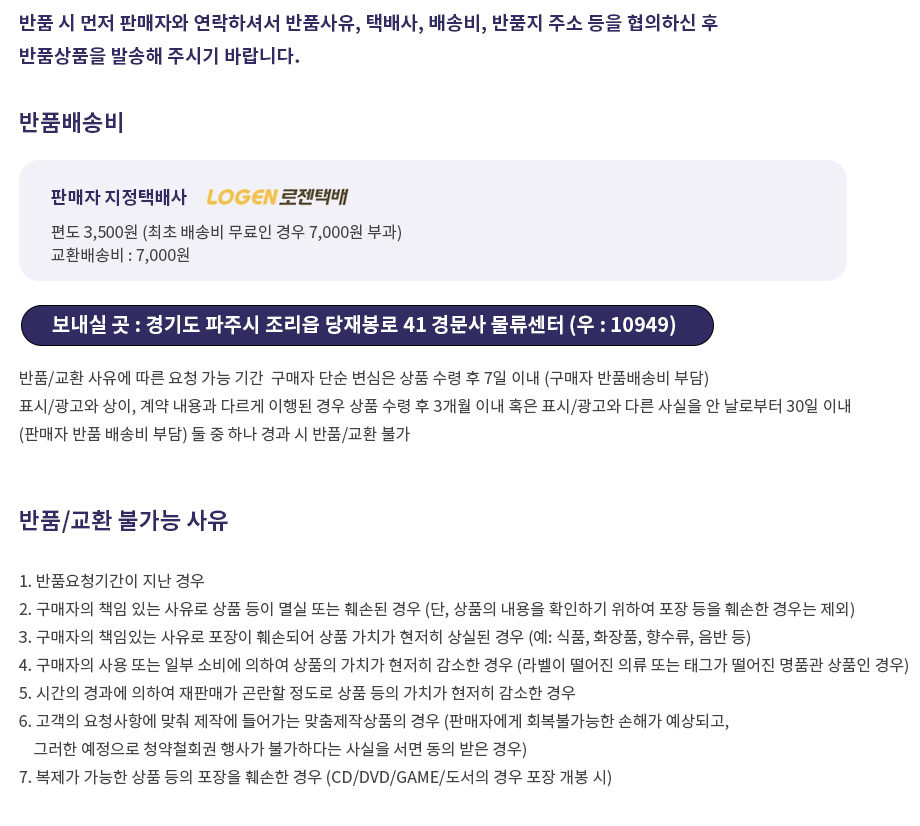## 로그인이필요합니다## 도서를 검색해 주세요.

원하시는 결과가 없으시면 문의 주시거나 다른 검색어를 입력해보세요.

## 상품간략정보 및 구매기능

Ordinary Differential Equations

상품 선택옵션 0 개, 추가옵션 0 개

지은이 W Cox 1996-01-05 222 9780340632031 구매가능 16,000원 0점 주문시 결제
• Ordinary Differential Equations
+0원
• Building on introductory calculus courses, this text provides a sound foundation in the underlying principles of ordinary differential equations. Important concepts, including uniqueness and existence theorems, are worked through in detail and the student is encouraged to develop much of the routine material themselves, thus helping to ensure a solid understanding of the fundamentals required. The wide use of exercises, problems and self-assessment questions helps to promote a deeper understanding of the material and it is developed in such a way that it lays the groundwork for further study of partial differential equations.

• 1 Introduction and a Look Ahead
2 First-order Differential Equations-Methods and Models
3 First-order Differential Equations-Analysis and Approximation
4 Second- and Higher-order Homogeneous Linear Differential Equations
5 Inhomogeneous Linear Differential Equations
6 Laplace Transform Methods for Solving Initial Value Problems
7 Systems of Linear Differential Equations
8 Series Solution of Linear Differential Equations
9 Special Functions and Orthogonal Expansions
10 An Introduction to Nunlinear System
Appendix - Chapter Summaries
Ansers to Exercises

• 학습자료

등록된 학습자료가 없습니다.

정오표

등록된 정오표가 없습니다.

사용후기가 없습니다.

상품문의가 없습니다.

• ## 배송/교환정보

### 배송정보### 교환/반품